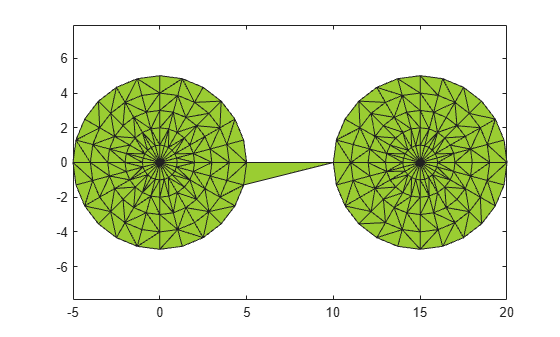criticalAlpha

Alpha radius defining critical transition in shape

Description

example

a = criticalAlpha(shp,type) returns the critical alpha radius that produces a notable transition in the alpha shape. Specifying type as 'all-points' returns the smallest alpha radius producing an alpha shape that encloses all points. Specifying type as 'one-region' returns the smallest alpha radius producing an alpha shape that encloses all points and has only one region.

Examples

collapse all

Create and plot a set of 2-D points.

th = (pi/12:pi/12:2*pi)';
x1 = [reshape(cos(th)*(1:5), numel(cos(th)*(1:5)),1); 0];
y1 = [reshape(sin(th)*(1:5), numel(sin(th)*(1:5)),1); 0];
x = [x1; x1+15;];
y = [y1; y1];
plot(x,y,'.')
axis equalCreate an alpha shape using the default alpha radius.

shp = alphaShape(x,y);

Compute the smallest alpha radius that produces an alpha shape enclosing all of the points and plot the corresponding alpha shape.

pc = criticalAlpha(shp,'all-points');
shp.Alpha = pc;
plot(shp)Compute the smallest alpha radius that produces an alpha shape enclosing all of the points and having only one region.

shp = alphaShape(x,y);
pc = criticalAlpha(shp,'one-region');
shp.Alpha = pc;
plot(shp)Input Arguments

collapse all

Alpha shape, specified as an alphaShape object. For more information, see alphaShape.

Example: shp = alphaShape(x,y) creates a 2-D alphaShape object from the (x,y) point coordinates.

Type of critical transition, specified as either 'all-points' or 'one-region'.

• 'all-points' corresponds to the smallest alpha radius producing an alpha shape that encloses all points.

• 'one-region' corresponds to the smallest alpha radius producing an alpha shape that encloses all points and has only one region.

Data Types: char

Output Arguments

collapse all

Critical alpha radius, returned as a scalar. a is the value of the alpha radius that produces an alpha shape, which either encloses all points (if type is 'all-points'), or encloses all points within a single region (if type is 'one-region').

After using criticalAlpha to find a, you can make the alpha radius of shp equal to a by typing shp.Alpha = a.